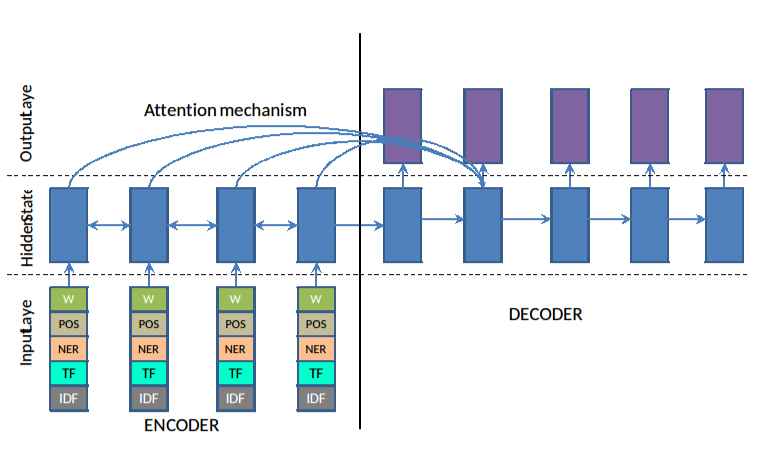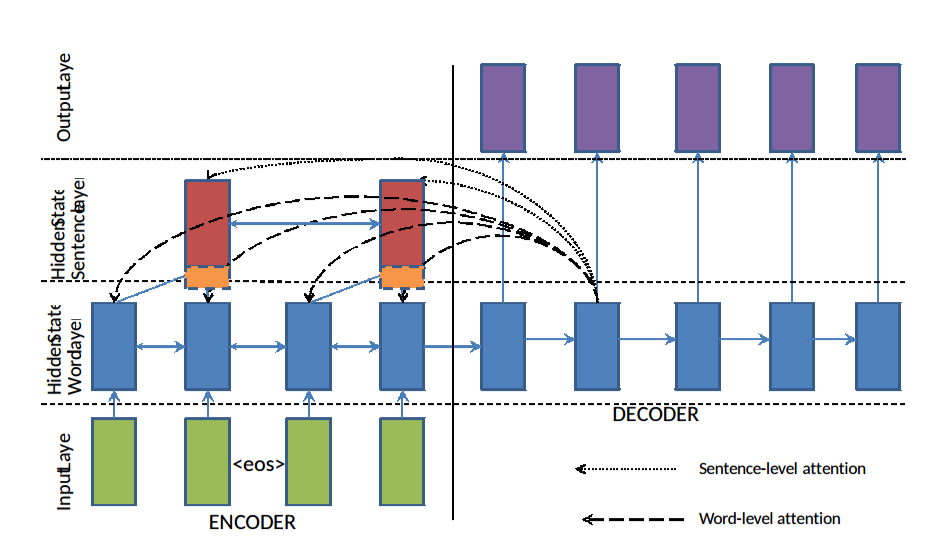### Paper - arXiv

#### Hypothesis

• Modeling abstractive text (sentence) summarization using Attentional Encode Decoder neural nets. Propose models for handling out of word vocabulary, hierarchical structure, and adding features in the encoder.

#### Interesting methods

• Based on the standard Encoder-Decoder framework with attention, they propose a few additional extensions.
1. Large Vocab Trick, instead of softmax over the entire vocabulary, softmax over the vocabulary from the source document as well as the top frequent words in the vocabulary.
2. Capturing Keywords using feature-rich Encoder: Concatenate the word vectors in the source with TF, IDF features as3- Handling Out of Vocabulary terms by pointing the decoder to a location in the source document instead of generating a new word. A switch at each time step decides whether to point to a source document word or generate a new word. It is modeled as a sigmoid function over a linear layer on the context at each time step.4- Hierarchical attentions for capturing hierarchical document structure: Two RNNs on the source side, one at word level and the other at the sentence level. Attention operates at both levels simultaneously. Word attention is re-weighted by the corresponding sentence-level attention and re-normalized as: $P^(a)(j)=\frac{P^a_w(j)P^a_s(s(j))}{\sum\levels_{k=1}^{N_d}P^a_w(k)P^a_s(s(k))}$ $P^a_w(j)$ is the word-level attention weight at $j^{th}$ position of the source document, $P^a_s(s(j))$ is the sentence-level attention weight for the sentence at the $j^{th}$ position. $N_d$ is the number of words in the source document, and $P^a(j)$ is the re-scaled attention at the $j^th$ word position.#### Results

• Gigaword, DUC, CNN/Daily Mail
• slight improvements over Rush et al. 2015